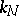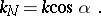# Meusnier theorem

Ifis a curve lying on a surface andis a point on, then the curvatureofat, the curvatureof the normal section of the surface by the plane passing through both the unit tangent vector toatand the unit normal vector to the surface, and the anglebetween the referred plane ofatand the osculating plane, satisfy the relation